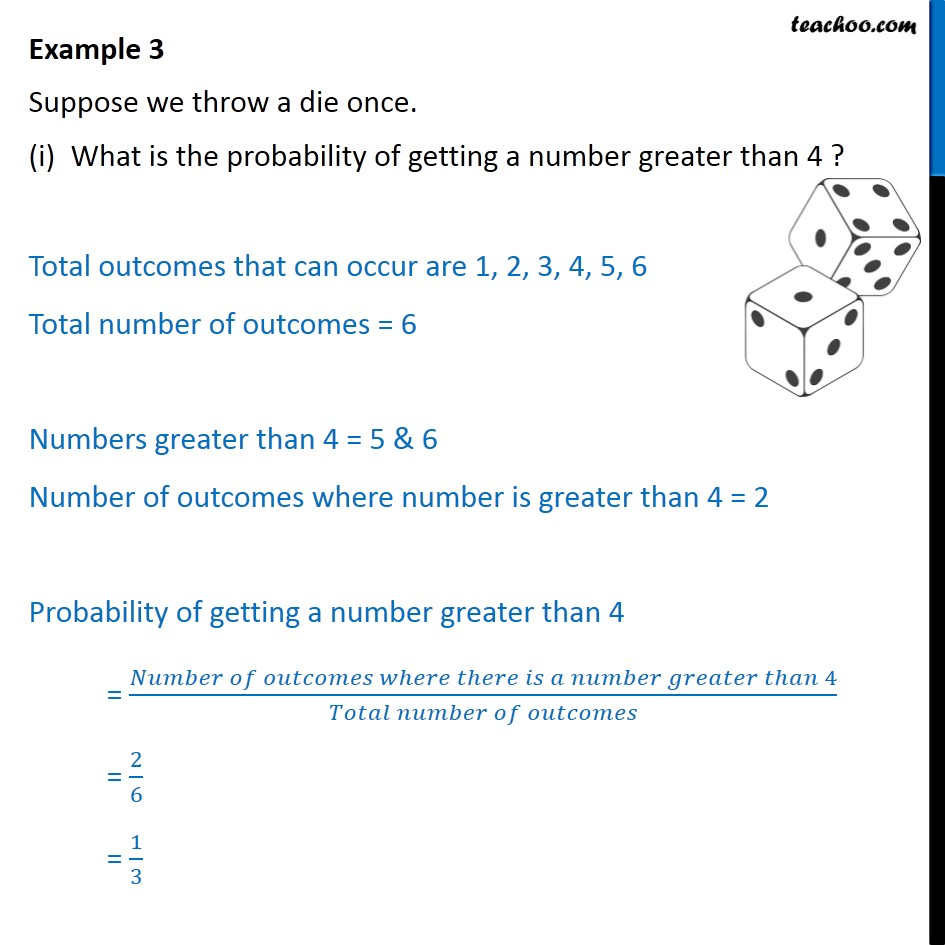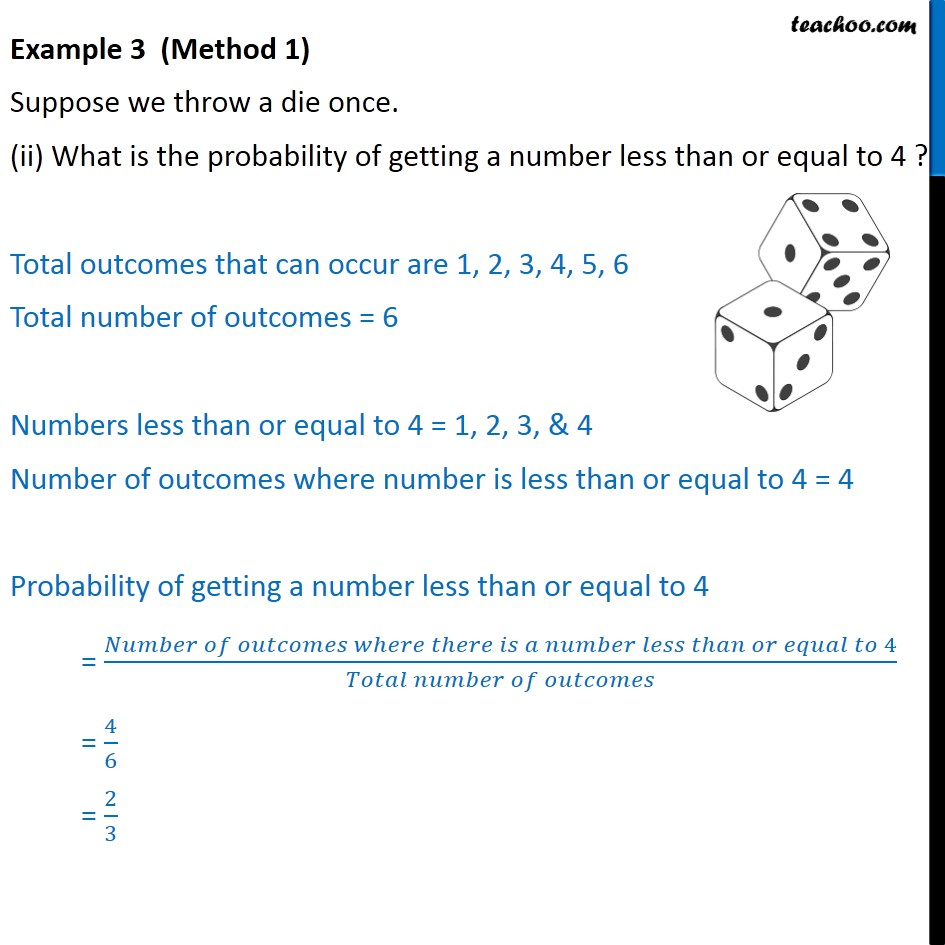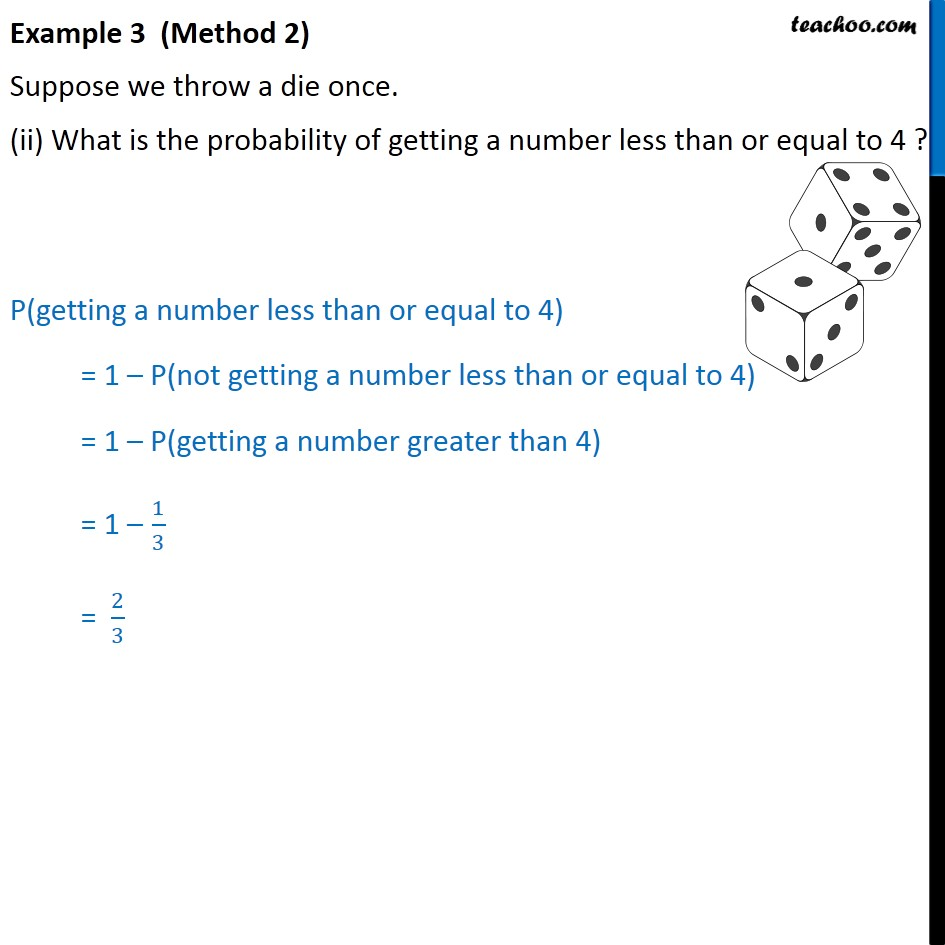Subscribe to our Youtube Channel - https://you.tube/teachoo

1. Chapter 15 Class 10 Probability
2. Concept wise
3. Die

Transcript

Example 3 Suppose we throw a die once. What is the probability of getting a number greater than 4 ? Total outcomes that can occur are 1, 2, 3, 4, 5, 6 Total number of outcomes = 6 Numbers greater than 4 = 5 & 6 Number of outcomes where number is greater than 4 = 2 Probability of getting a number greater than 4 = (𝑁𝑢𝑚𝑏𝑒𝑟 𝑜𝑓 𝑜𝑢𝑡𝑐𝑜𝑚𝑒𝑠 𝑤ℎ𝑒𝑟𝑒 𝑡ℎ𝑒𝑟𝑒 𝑖𝑠 𝑎 𝑛𝑢𝑚𝑏𝑒𝑟 𝑔𝑟𝑒𝑎𝑡𝑒𝑟 𝑡ℎ𝑎𝑛 4)/(𝑇𝑜𝑡𝑎𝑙 𝑛𝑢𝑚𝑏𝑒𝑟 𝑜𝑓 𝑜𝑢𝑡𝑐𝑜𝑚𝑒𝑠) = 2/6 = 1/3 Example 3 (Method 1) Suppose we throw a die once. (ii) What is the probability of getting a number less than or equal to 4 ? Total outcomes that can occur are 1, 2, 3, 4, 5, 6 Total number of outcomes = 6 Numbers less than or equal to 4 = 1, 2, 3, & 4 Number of outcomes where number is less than or equal to 4 = 4 Probability of getting a number less than or equal to 4 = (𝑁𝑢𝑚𝑏𝑒𝑟 𝑜𝑓 𝑜𝑢𝑡𝑐𝑜𝑚𝑒𝑠 𝑤ℎ𝑒𝑟𝑒 𝑡ℎ𝑒𝑟𝑒 𝑖𝑠 𝑎 𝑛𝑢𝑚𝑏𝑒𝑟 𝑙𝑒𝑠𝑠 𝑡ℎ𝑎𝑛 𝑜𝑟 𝑒𝑞𝑢𝑎𝑙 𝑡𝑜 4)/(𝑇𝑜𝑡𝑎𝑙 𝑛𝑢𝑚𝑏𝑒𝑟 𝑜𝑓 𝑜𝑢𝑡𝑐𝑜𝑚𝑒𝑠) = 4/6 = 2/3 Example 3 (Method 2) Suppose we throw a die once. (ii) What is the probability of getting a number less than or equal to 4 ? P(getting a number less than or equal to 4) = 1 – P(not getting a number less than or equal to 4) = 1 – P(getting a number greater than 4) = 1 – 1/3 = 2/3

Die

About the AuthorDavneet Singh
Davneet Singh is a graduate from Indian Institute of Technology, Kanpur. He has been teaching from the past 9 years. He provides courses for Maths and Science at Teachoo.